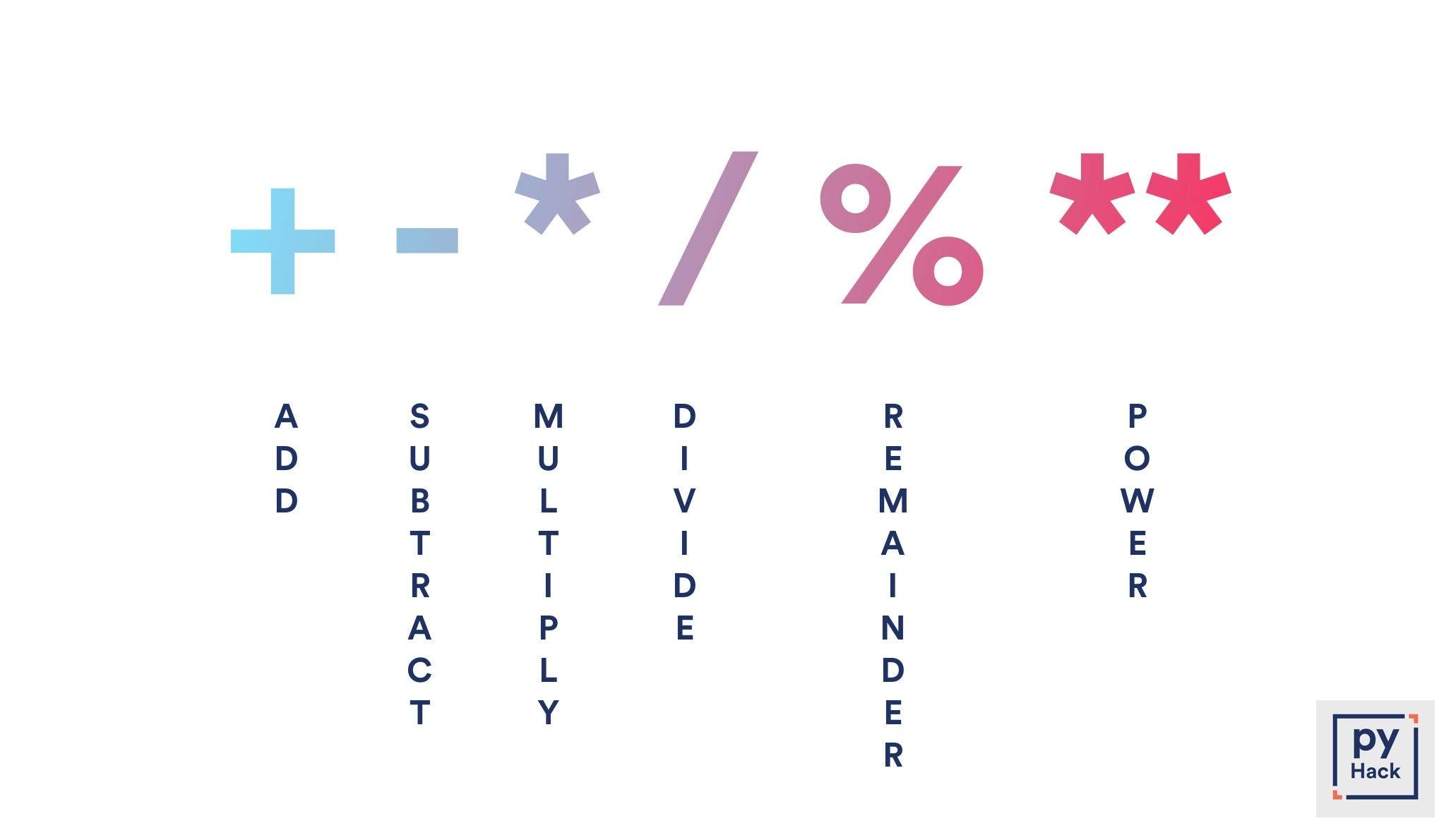# Python Numbers in under 10 minutes

## Learn the basics of the Python Data type Numbers

Every value that we work with in Python has a type. A data type is a classification that specifies which type of value a variable has and what type of operations can be applied to it. It’s as simple as that. This article is a quick introduction to the Numbers data type in python. So let’s get started!

▬▬▬▬▬▬▬▬▬▬▬▬▬▬▬▬▬▬▬▬▬▬▬▬▬▬▬▬▬▬▬▬

# Numbers

Numbers are the most basic data types in Python. Any number that you assign to a variable is of the data type ‘Number’.

Now within Numbers, there are three classes :

1. Integer
2. Floating point numbers
3. Complex Numbers

These are the types of Numbers Python supports.

▬▬▬▬▬▬▬▬▬▬▬▬▬▬▬▬▬▬▬▬▬▬▬▬▬▬▬▬▬▬▬▬

# 1. Integer (int) :

Any and every whole number falls in ‘`int`’ class. Any number without decimals is an Integer.

`myVar1 = 20myVar2 = 10000myVar3 = 0`

Let’s quickly check the type of the above variables using the` type()` method.

Run the code by hitting the big green play button. You can also edit the code and run it as well.

If you run the code above by clicking on the big green play button, you’ll get an output saying these variables are of `<class ‘int’>`. This tells us the type(class) the variable belongs to.

▬▬▬▬▬▬▬▬▬▬▬▬▬▬▬▬▬▬▬▬▬▬▬▬▬▬▬▬▬▬▬▬

# 2. Floating Point Numbers (float) :

All decimal numbers fall under the ‘float’ class. Any number with decimals is of a float type. The result of a division operation between two numbers results in a floating-point number. Even if the result is a whole number it will be converted to floating-point.

`myVar = 1.2345myVar2 = 1231.232323myVar3 = 6/3  # Even though the result is 3 which is a whole number, it will be be converted to a floating point number resulting in the value 3.00`

Let’s quickly run the above code:

If you run the code above, you’ll get an output saying these variables are of the `<class ‘float’>`.

▬▬▬▬▬▬▬▬▬▬▬▬▬▬▬▬▬▬▬▬▬▬▬▬▬▬▬▬▬▬▬▬

# 3. Complex Numbers (complex) :

Complex numbers are specified as `<real part> + <imaginary part>j`. Just like how you would do in regular math. But this type is rarely used.

`myVar = 2 + 3jmyVar2 = 6 + 3jprint(type(myVar))print(type(myVar2))print(myVar + myVar2)`

Run the code below!

If you check the type of the above variable, it’ll return `<class ‘complex’>`.

▬▬▬▬▬▬▬▬▬▬▬▬▬▬▬▬▬▬▬▬▬▬▬▬▬▬▬▬▬▬▬▬

# Operations on Numbers:

We can perform the basic mathematical operations on numbers using what is known as an “operator”. It’s nothing but a symbol that defines what type of operation you want to perform on numbers. You can use these operators to perform operations on variables containing numbers.Gotta love gradients

The `+` operator is used for addition.

`myVar1 = 1 + 2myVar2 = 3 + 5myVar3 = myVar1 + myVar 2`

The `-` operator is used for subtraction.

`myVar1 = 1 - 2myVar2 = 3 - 5myVar3 = myVar1 - myVar 2`

The `*`operator is used for multiplication.

`myVar1 = 1 * 2myVar2 = 3 * 5myVar3 = myVar1 * myVar 2`

The `/` operator is used for division.

`myVar1 = 4 / 2myVar2 = 2 / 1myVar3 = myVar1 * myVar 2`

The `%` operator is used to find the remainder of a division operation.

`myVar = 6 % 4# Which will give you 2.`

The `**` operator is used to raise the number on the left side of the operator to the power of the number on the right side of the operator.

`myVar = 2 ** 3myVar2 = 2 ** 2myVar3 = 3 ** 3`

Okay so now let’s run the code on a repl portal and see the results.

You can also use `()` to separate multiple mathematical operations just like you would on a calculator.

`myVar = (1 + 2) * (3 / 4)myVar = (3 * 4) / (6 / 3)myVar = ((3 * 4) / (6 / 3) - (1 + 2) * (3 / 4))# And so on ...# You get the point. Right?`

If you’ve reached till here, GOOD JOB! You’ve taken your first steps into learning Python. In this post we learned about the Numbers Data Type, it’s subtypes and the operations you can perform on it. In the next post, we’ll learn more about strings.

# And that’s pretty much it for the Numbers Data Type in Python. What? I’m serious. This is all you need to know about numbers.

▬▬▬▬▬▬▬▬▬▬▬▬▬▬▬▬▬▬▬▬▬▬▬▬▬▬▬▬▬▬▬▬

# Peace Out ✌️

▬▬▬▬▬▬▬▬▬▬▬▬▬▬▬▬▬▬▬▬▬▬▬▬▬▬▬▬▬▬▬▬

I write about Tech. Algorithms. Programming. And that kinda thing you know?

I write about Tech. Algorithms. Programming. And that kinda thing you know?